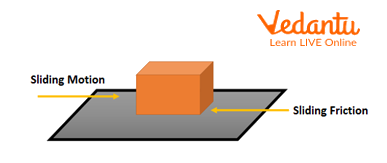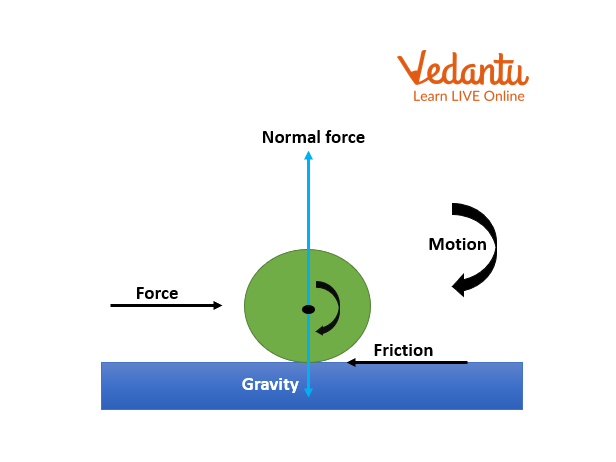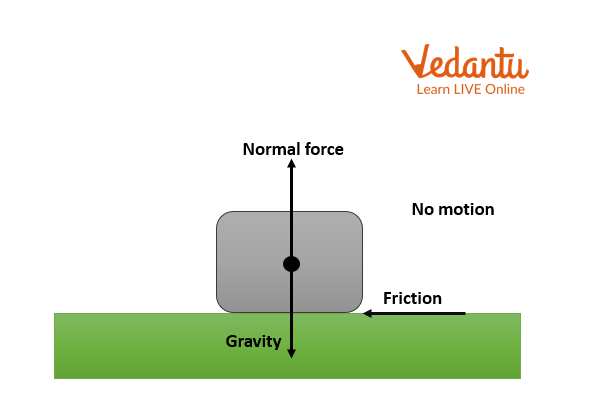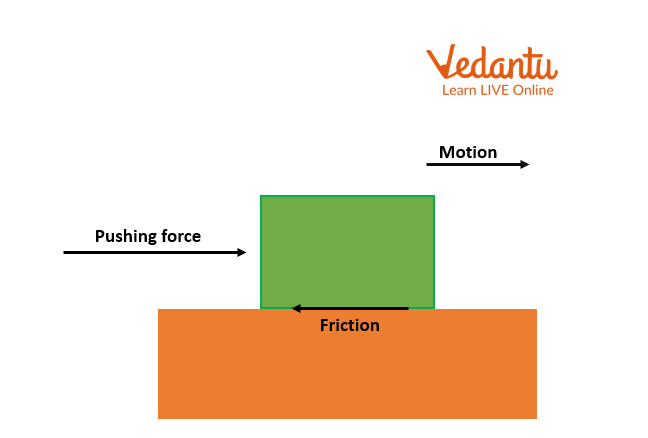Courses
Courses for Kids
Free study material
Offline Centres
More

# Relationship Between Weight of a Body and ForceLast updated date: 26th Nov 2023
Total views: 12.9k
Views today: 0.12k## To Determine the Relationship Between Friction and Force

### Introduction

When two surfaces move across one another or attempt to move across one another, friction is a force that is produced and is directly proportional to the weight of the body. The motion is always opposed by friction when the two surfaces come in contact with each other and prevents an object from moving. The frictional force keeps our feet firmly planted on the ground as we walk on the ground or a floor as well as when we run on a surface.

• Aim

• Theory

• Procedure

• Observations

• Results

### Aim

With a spring balance, creating a relationship between the weight of a rectangular wooden block lying horizontally and the least amount of force necessary to simply move it.

### Apparatus Required

• A spring balance

• a bench

• a wooden slab with slots of weights

• a string

• slotted weights

## Theory

Every time a force is applied, frictional force always operates in the opposite direction. As more power is exerted on the wooden block to be moved, more friction is created to keep it balanced. This is an indication of static friction or friction that occurs when an object is at rest.Experimental Demonstration of the Static Friction

However, there is a limit to how much the force of friction can grow. When the applied force reaches this threshold, the object starts to move. The limiting force of friction, also known as limiting friction, is the greatest amount of force of friction that acts between two solid surfaces right before an object begins to move. The weight of the thing has a direct relationship to the force.

### Procedure

1. Find the spring balance's range and least count.

2. Using a spring balance, measure the wooden block's weight.

3. As indicated, place a wooden block on the horizontal surface of the setup and attach it to a spring balance.

4. Check the spring balance's pointer.

5. Now pull the spring balance to exert a slight strain on the wooden block. Increase the force gradually until the block starts to slide.

6. Take note of the spring balance's reading.

7. Repeat the experiment using a wooden block that is 50 g heavier each time, and note your findings.

### Observations

1. The spring balance's operating range =

2. The least count of spring balance =

3. Mass of the hook-shaped wooden block =

4. Value of the gravitational acceleration g =

5. The spring balance's least count, = 10 g

6. Mass of the hook-shaped wooden block, R= 50 g

## Observation Table

### \[\dfrac{F}{W}\]

1

0

2

50 g

3

100 g

4

150 g

5

200 g

Plot a graph between the spring balance reading, F on the 'y' axis, and the weight of the wooden block, W, on the x-axis. A straight line will form.

## Results

1. It was found that the force required to be increased as the block's weight did as well.

2. \[\dfrac{F}{W}\] is a constant ratio.

3. The maximal force needed to simply move the block is precisely proportional to the weight of the block, as seen by the constant \[\dfrac{F}{W}\] ratio and the straight-line graph.

## Precautions

• As soon as the wooden block begins to move, it is important to take note of the reading in spring balance.

• The horizontal surface needs to be dry and clean.

• The wall or any other surface should not be touched by the spring balance.

• When tugging the string, the amount of force that is given to the wooden block should be raised gradually and gently.

• The string must not extend further.

• When applying force to the block, the string must remain horizontal.

## Lab Manual Questions

1. Why are the wooden block you are using, and the thread used to move it both kept horizontal?

Ans: The body is dragged horizontally because we must slide it across the surface to get the frictional forces.

2. In which direction does the frictional force affect the block?

Ans: The direction of the block's motion is opposed by the force of friction.

3. How does adding grease or oil between two surfaces in contact influence the limiting friction between them?

Ans: Fluids are applied to a surface, which smooths it out by reducing friction and the force needed to move an object.

4. What is the meaning of 1 gwt measured in your experiment?

Ans: 1 gwt is defined as the force exerted by the mass of 1 gm due to the gravity, which is basically given as mg and equals 0.098 N.

## Viva Questions

1. What is the SI unit of friction?

Ans: Newton (N).

2. Why don't wooden blocks move when they are first placed?

Ans: Due to the static friction, the wooden block does not move on the surface and only moves when a force greater than the static friction will be applied on the wooden block.

3. Why do wooden blocks begin to move after receiving a certain level of force?

Ans: Wooden pieces begin to move once when the applied force exceeds the limit of the static friction force.

4. What Newtonian law does friction comply with?

Ans: Third law of Newton (Law of action and reaction).

5. Why is it advised to clean the tables before conducting experiments?

Ans: Because of the dust present on the table surface which increases the friction of the surface.

6. Why do we refer to friction as an adjustable force?

Ans: Friction is zero when no force is applied to the item. The force of static friction is always equal to the applied force until the block starts moving, therefore as the applied force grows, friction correspondingly increases. Static friction thus adjusts itself in the direction opposite to the applied force.

7. Why are the bodies of cars, planes, and launch vehicles for spacecraft made in a streamlined shape?

Ans: They are specifically shaped in a streamlined manner to reduce the impact of air friction and therefore the efficiency of operation increases.

8. Why does moving a heavier object involve more effort than moving a lighter object?

Ans: Normal reaction force 'N' also rises for a heavy body. As a result, frictional force grows. Consequently, more force is applied.

9. Why are luggage bags equipped with rollers?

Ans: Since the sliding friction is greater in magnitude as compared to the rolling friction and therefore to pull the heavier bags they are equipped with the rollers.Sliding MotionRolling Friction

10. What are the variables that determine how much force is needed to simply move over the surface of a body while another surface is in contact?

Ans: It depends on the type of material used for the surfaces coming into contact as well as how smooth or rough those surfaces are.

## Practical-Based Questions

1. A table is being pushed downward by a block resting on it. This force's response is

1. Earth’s force on the block

2. The tension on the block caused by the table

3. Earth’s force on table

4. The block's force on Earth

Ans: B) The tension on the block caused by the table

2. The “action” force remains in effect despite the “reaction” force because

1. The force of the action exceeds that of the reaction.

2. All are moving in the same direction

3. They have an impact on the two various bodies in contact.

4. Once the action force is gone, the reaction force only exists.

Ans: C) They have an impact on the two various bodies in contact.

3. On a horizontal surface, an object travelling to the right is subjected to a force F that is greater than the maximum force of static friction. Then

1. There must be a constant rate of motion for the object.

2. Force acts in the opposite direction from the friction force.

3. The object must be moving more quickly.

4. The object must be slowing down.

Ans: B) Force acts in the opposite direction from the friction force.

4. The table's surface is horizontal, and a wooden block is laying there. The forces affecting this wooden block are

1. Movement on the table's surface

2. Reaction happening on the wooden block

3. Motion on the table's surface and a reaction on the wooden block

4. None of the above

Ans: C) Motion on the table’s surface and a reaction on the wooden block

5. Frictional force acting on an object resting on a rough surface, will be

1. Static friction

2. Kinetic friction

3. Rolling friction

4. Sliding friction

Ans: A) Static friction

6. How much force must be used in order to move an object at a constant speed?

1. No force is necessary.

2. Force must be greater than the force of friction.

3. Force must be smaller than the force of friction.

4. Just enough force to equal the frictional force.

Ans: B) Force must be greater than the force of friction

7. When an object is subject to a balanced set of forces, then

1. At rest

2. Moving.

3. Must not be speeding up.

4. None of the above

Ans: C) Must not be speeding up.

8. Fish's streamlined bodies make it easier for them to

1. easily swim.

2. lessen the friction

3. increase the friction.

4. Both A and B

Ans: B) Both A and B

9. On ice, skating is simple, but moving on concrete is challenging because its coefficient of friction is

1. higher on ice rather than concrete

2. higher on concrete rather than ice.

3. similar in both.

4. distinct from an usual reaction

Ans: B) Higher on concrete rather than ice

10. The spring balance that is used to determine the least amount of force required to simply slide a wooden block has a least count of 1 gwt. what will be the number of divisions between its 50 gwt and 60 gwt marks?

1. 60

2. 20

3. 10

4. 50

Ans: C) 10

## Conclusion

A force known as friction prevents relative motion between surfaces that are in touch. One of the more basic features of friction is that it always runs parallel to the surfaces with which it makes contact and opposes any motion or attempted motion of the systems with respect to one another. From this article, we can understand the causes affecting friction, types of friction with some examples.

## FAQs on Relationship Between Weight of a Body and Force

1. What are the different types of frictions based on the state of motion of the object?

Static and kinetic friction. The form of friction that exists between a stationary object and the surface it is placed on is known as static friction. When we position something somewhere, it prevents it from moving around. It is seen as the force that acts between moving surfaces is known as kinetic friction. A force acting in the opposite direction of the movement of a body on the surface is felt.Static FrictionKinetic Friction

2. How does adding grease or oil between two surfaces in contact influence the limiting friction between them?

The roughness of the surfaces in contact is responsible for the frictional force, which can be microscopic and macroscopic. When the fluids like grease or oil are applied to a surface, which smooths it, i.e., reduces the roughness of surfaces in contact and therefore reduces the friction and hence the force needed to move an object.

3. How can you measure a force using the idea of limiting friction?

When an object is placed on a surface, the frictional force created by the surface prevents it from moving, even when force is applied. The item simply overcomes the frictional effect and moves when the applied force is unbalanced. By doing so, we may calculate the limiting friction.

4. What are the different categories of friction?

In general the type of friction is defined in three ways, (i) on the basis of state of motion, that are static and kinetic, (ii) on the basis of contact between surfaces, that are rolling and sliding, and (iii) on the basis of matter subject of object, that are air and fluid. These frictions are different in magnitudes.

5. What can be the reason behind increasing the friction purposefully?

In some situations, like the grooves in shoe soles, the treaded tyres of cars, trucks, or other vehicles, or the use of brake pads in the brake system of bicycles and cars, we need to increase friction. If we don't, it all might slip on the ground or on the roads, thus causing a high chance of accidents.